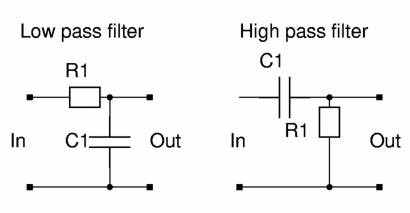Returning to low pass filters

# Soil Moisture Sensor Project

## Returning to low pass filters

Having reached the limits of Clapp oscillators, it was time to look again at the methods I had initially rejected.  I originally rejected low pass filters because the designs required relatively expensive components or ran at low frequencies. Apart from the cost, they are relatively simple because they consist of three parts:

OSCILLATOR -> LOW PASS FILTER -> SIGNAL STRENGTH MEASUREMENT

Each of these three parts can use a capacitor. But as we have seen, it is not feasible to use a soil capacitor in the oscillator. But what if we had an oscillator that ran at a high frequency and tried to use soil as a capacitor in one of the other places…. (the low pass filter or signal strength measurement). Let’s look at each of these places.

### Low Pass Filter

As we saw earlier, a simple filter is made of a resistor and a capacitor. (Have a quick re-read of that page if you want to learn about how the filter works, otherwise “How Stuff Works” is a great resource”)

Just as revision: In a filter, the capacitor acts like a resistor. The value of its resistance changes according to the frequency that is passing through it. This means that you effectively have two resistors in series… which is a voltage divider. But in this voltage divider, no worms get electrocuted.When soil is used as the capacitor in a low pass filter, the moisture level of the soil determines how much the signal is weakened. The more moist the soil, the greater the capacitance, and the weaker the signal will become. We can measure the strength of this signal to determine the value of the capacitor.

### Signal Strength Measurement

Another area that can use a capacitor is a “diode detector”. Unfortunately a diode detector can also be known as a “peak detector”, an “envelope detector”, or an “AM demodulator”.

This schematic shows a very simple diode detector. Below the schematic is a diagram showing what happens to a radio signal in the detector. The “radio frequency signal” is made up of two separate frequencies. The first is a low frequency (two peaks in the diagram below the schematic) and the second is a carrier higher frequency (20 peaks). The blue line represents zero volts and the radio signal alternates above and below zero volts. “Modulation” is the process of changing the voltage (also known as amplitude) of the higher frequency. The lower frequency is combined with the higher frequency which changes the amplitude of the higher frequency signal. This creates an “Amplitude Modulation” signal (the AM section of your radio that plays talkback radio and music from many decades ago).

You have used a simple diode detector if you built a crystal radio. The “radio frequency signal” came from the coil of wire (and perhaps a variable capacitor) you used to tune the antenna. You still had a diode which is the most important part of the crystal radio and diode detector. Instead of a resistor and capacitor you had an ear piece through which you listened to… what ever that language was. For more information, this StackExchange page is great.

### How a diode detector works

We are interested in the “demodulated signal”. The signal goes through the diode at the top of the schematic (the symbol for the diode is the triangle pointing into a rectangle). The diode only lets electrical current flow in one direction, which is the process of “rectifying” the signal. In this case, the negative voltages are removed from the radio signal.

The “rectified signal” then charges up the capacitor. If we kept the negative voltages, then they would discharge the capacitor immediately after the positive voltage charged it up. The capacitor slowly discharges through the resistor. When we measure the voltage across the resistor and capacitor we will see it rise as the amplitude of the radio signal increases, and fall as the amplitude decreases.

This section was a very long way of saying that we are undoing all of the work that went in to varying the amplitude of the higher frequency signal.

Another good resource if you want to understand how the diode detector works are the answers to a question about a diode detector on StackExchange.

### What would happen if we used a variable capacitor in a diode detector?

Good question. I could not find any examples of this approach when trawling Google searches. I suspect that as the capacitance increases, the voltage across the resistor and capacitor would decrease because it would take more to charge up a larger capacitor. If the capacitance decreases I think that the voltage would increase.

Perhaps I’ll give it a go.

Go back to the main page,